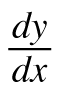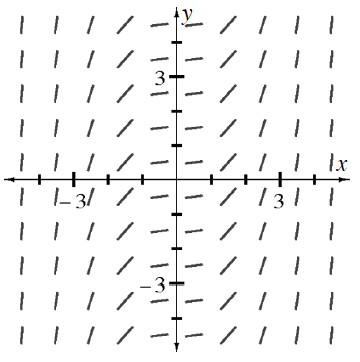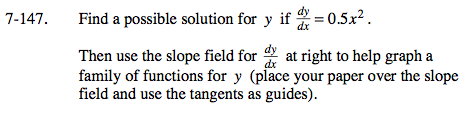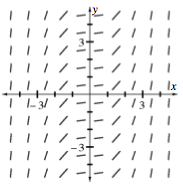### Home > CALC > Chapter 7 > Lesson 7.3.4 > Problem7-147

7-147.

Find a possible solution for y if= 0.5x2.
Then use the slope field forbelow to help graph a family of functions for y (place your paper over the slope field and use the tangents as guides). Homework Help ✎By tracing a series of slopes, you should predict that the original function is cubic.Separate the variables so that x-values are on one side of the equal sign and y-values are on the other side.

Integrate both sides of the equation.

Solve for y... Did you get a cubic function, as predicted?
Note: C1 + C2 = C3, or just C.

2dy = x2dx

$\int 2dy=\int x^{2}dx$

$2y+C_{1}=\frac{x^{3}}{3}+C_{2}$